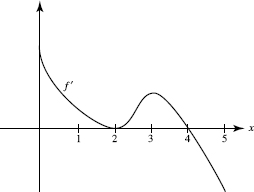# AP Calculus AB Practice Test 29

### Test Information8 questions27 minutes

Calculator Allowed

1. If F(3) = 8 and F (3) = -4 then F(3.02) is approximately

2. An object moving along a line has velocity v(t) = t cos t - ln (t + 2), where 0 ≤ t ≤ 10. How many times does the object reverse direction?

3. Refer to the graph of f below.f has a local minimum at x =

4. Refer to the graph of f below.The graph of f has a point of inflection at x =

5. For what value of c on 0 < x < 1 is the tangent to the graph of f (x) = ex - x2 parallel to the secant line on the interval [0,1]?

6. Find the volume of the solid generated when the region bounded by the y-axis, y = ex, and y = 2 is rotated around the y-axis.

7. The table below shows the "hit rate" for an Internet site, measured at various intervalsduring a day. Use a trapezoid approximation to estimate the total number of peoplewho visited that site.

 Time Midnight 6 A.M. 8 A.M. Noon 5 P.M. 8 P.M. Midnight People perminute 5 2 3 8 10 16 5

8. The acceleration of a particle moving along a straight line is given by a = 6t. If, when t = 0, its velocity, v, is 1 and its position, s, is 3, then at any time t